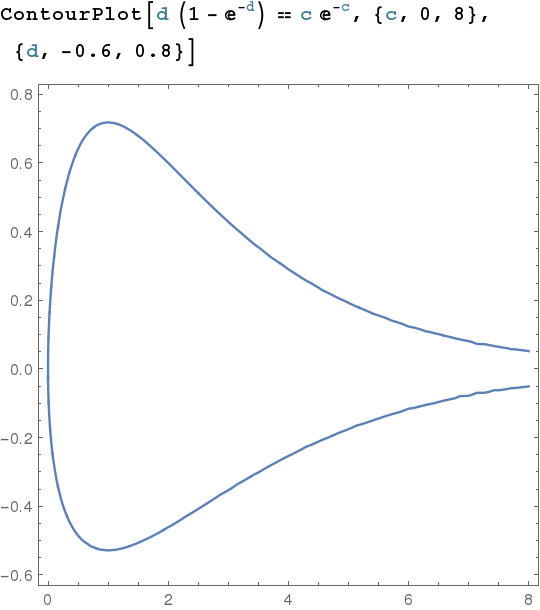# Integro-differential equation

I have an equation of the type $$f-\frac{\sigma^2} 2 \frac{d^2 f}{dx^2}-\frac{df}{dx} = \int_0^\infty \left(\frac{df}{dx}\right)^2\exp(ax+f(x)) \, dx.$$

It is an integro-differential equation but the integral is on the fixed domain $[0;\infty[$ independent of $x$. I know we use the Laplace transform for integro-differential equations in some cases. Here I'm trying to rewrite the equation in a simple way. If that fails I would be happy with a numerical method that can deal with it. Usual ODE solvers I tried could not solve it numerically because of the unknown appearing in the integral.

• You can assign an arbitrary value $c\geq0$ to the integral in the right-hand side. Simply use the boundary condition $f(0) + f'(0)/\kappa = c$ (see my answer below). This problem is always solvable, and you get up to three solutions (depending on $a$, $\kappa$, and $c$). – ifw Jan 29 '17 at 16:55

Replace the right side by constant $c$. The d.e. $f - \frac{\sigma^2}{2} f'' - f' = c$ can be solved explicitly. Then you have an equation to solve: $c = \int_0^\infty f'(x)^2 \exp(ax + f(x))\; dx$.

EDIT: With a nonlinear term, there is the real possibility that the solution will blow up at some finite $t$; even without it, the integral may diverge. But if you can identify a region where everything converges nicely, you can use a numerical equation-solver where the integral is evaluated numerically using a numerical solution of the differential equation.

• Thank you. I did this but I kind of simplified the actual problem in my exposition here. On the left hand side of my equation there is actually also a term in $(f')^2$ which makes the explicit solution not an option. If I were to solve it numerically I would have to make a guess for $c$ find $f$ and check whether the equation of $c$ is satisfied. I wonder if there is another way. – skillfeedback Jan 23 '17 at 18:39
• You also haven't stated the initial conditions. It sometimes helps to state the actual problem rather than a problem you're not interested in solving. – Robert Israel Jan 23 '17 at 20:54

Contrary to the OP's expectation, the integro-differential equation - henceforth referred to as (#) - can be solved explicitly. It is natural to prescribe the value of $f'(0)$ which is the boundary condition I'll consider below. As an effect of the nonlinearity, one then has two, one, or zero solutions.

It is convenient to work with the parameter $\kappa>0$, where $\sigma^2/2 = (\kappa+1)/\kappa^2$ (i.e., $\kappa=\left(1+\sqrt{1+2\sigma^2}\right)/\sigma^2$).

The result is as follows:

1. All solutions $f$ of (#) are of the form $$f(x) = c - d e^{-\kappa x},$$ where $c\geq0$ and $d\in\mathbb R$ are subject to the condition $$\Phi(d) = \Psi(c) \tag{*}$$ with $\Phi$ (depending on $a$ and $\kappa$) and $\Psi$ being entire functions (see below). If $f$ is a solution , then $c$ is the value of the integral in the right-hand side of (#).

2. There are three critical values $a_c$ (depending on $\kappa$), $0$, and $2\kappa$ for $a$ such that the following holds:

• For $a\leq a_c$, there is a $d^-<0$ (depending on $a$ and $\kappa$) such that (#) is solvable if and only if $f'(0)/\kappa\in[d^-,\infty)$.

• For $a_c<a<0$, there are $d^-<0<d^+<d^{++}$ such that (#) is solvable if and only if $f'(0)/\kappa\in[d^-,d^+]\cup [d^{++}, \infty)$

• For $0\leq a<2\kappa$, there are $d^-<0<d^+$ such that (#) is solvable if and only if $f'(0)/\kappa\in[d^-,d^+]$.

• For $2\kappa\leq a$, (#) is unsolvable.

Note. $a_c<0$ is implicitly determined by the condition that the (overdetermined) system $\Phi(d)=e^{-1}$ and $\Phi'(d)=0$ has a solution $d>0$.

Proof (sketch).

1. First one has to solve the ordinary differential equation $f(x) - \frac{\kappa+1}{\kappa^2}\,f''(x) -f'(x)= c$ on $[0,\infty)$ for a constant $c\geq0$. The bounded solution with $f'(0)/\kappa =d$ is $f(x) = c - d\,e^{-\kappa x}$. Then one is left with the integral equation $$\kappa^2 d^2 e^c \int_0^\infty e^{-d\,e^{-\kappa x}+ax-2\kappa x}\,dx = c.$$ The integral in the left-hand side converges precisely when $a<2\kappa$ and can be done explicitly. The result is (substitute $y= d\,e^{-\kappa x}$ if $d>0$, then use analytic continuation): $$\underbrace{\kappa\,d^{\,a/\kappa}\,\gamma\left(2-\frac{a}\kappa,d\right)}_{\Phi(d)} = \underbrace{c\,e^{-c}}_{\Psi(c)},$$ where $\gamma(s,x) = \int_0^x y^{s-1} e^{-y}\,dy$ is the lower incomplete Gamma function. Note that the function $x^{-s}\,\gamma(s,x)$ for $s>0$ (in our case $s=2-a/\kappa$) equals $\int_0^1 y^{s-1}e^{-x y}\,dy$ which is an entire function of $x$.

2. I'll only discuss the case $0<a<2\kappa$. In this case, one can show that $\Phi$ is strictly decreasing from $\infty$ to $0$ on the interval $(-\infty,0]$ and strictly increasing from $0$ to $\infty$ on the interval $[0,\infty)$, while $\Psi$ is strictly increasing from $0$ to $e^{-1}$ on the interval $[0,1]$ and strictly decreasing from $e^{-1}$ to $0$ on the interval $[1,\infty)$. Denoting by $d^-< 0 < d^+$ the two solutions $d$ of the equation $\Phi(d) = e^{-1}$, then there arise the following four cases:

• for $d=0$ one has $c=0$,
• for $d=d^-$ or $d=d^+$ one has $c=1$,
• for $d\in (d^-,0)\cup (0,d^+)$ there are two solutions $c$ of (*),
• for $d\in (-\infty,d^-)\cup (d^+,\infty)$ there is no solution $c$ of (*).

Example. For $a=1$, $\kappa=1$ (i.e., $\sigma=2$), (*) becomes $d\left(1-e^{-d}\right) =c \,e^{-c}$. Here is a plot of this relation:($d^-=-0.528399$ and $d^+=0.718078$.)